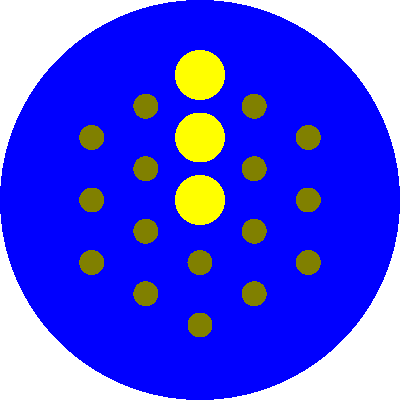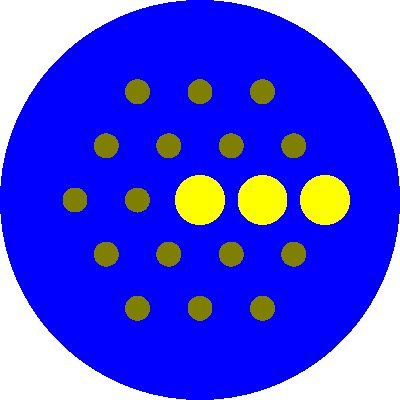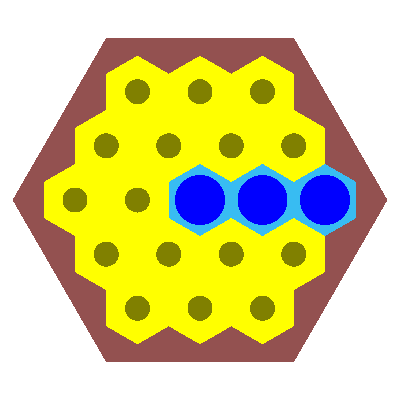# Modeling Hexagonal Lattices¶

hexagonal-lattice

In this example, we will create a hexagonal lattice and show how the orientation can be changed via the cell rotation property. Let's first just set up some materials and universes that we will use to fill the lattice.

In :
%matplotlib inline
import openmc

In :
fuel = openmc.Material(name='fuel')
fuel.set_density('g/cm3', 10.0)

fuel2 = openmc.Material(name='fuel2')
fuel2.set_density('g/cm3', 10.0)

water = openmc.Material(name='water')
water.set_density('g/cm3', 1.0)

mats = openmc.Materials((fuel, fuel2, water))
mats.export_to_xml()


With our three materials, we will set up two universes that represent pin-cells: one with a small pin and one with a big pin. Since we will be using these universes in a lattice, it's always a good idea to have an "outer" universe as well that is applied outside the defined lattice.

In :
r_pin = openmc.ZCylinder(r=0.25)
fuel_cell = openmc.Cell(fill=fuel, region=-r_pin)
water_cell = openmc.Cell(fill=water, region=+r_pin)
pin_universe = openmc.Universe(cells=(fuel_cell, water_cell))

r_big_pin = openmc.ZCylinder(r=0.5)
fuel2_cell = openmc.Cell(fill=fuel2, region=-r_big_pin)
water2_cell = openmc.Cell(fill=water, region=+r_big_pin)
big_pin_universe = openmc.Universe(cells=(fuel2_cell, water2_cell))

all_water_cell = openmc.Cell(fill=water)
outer_universe = openmc.Universe(cells=(all_water_cell,))


Now let's create a hexagonal lattice using the HexLattice class:

In :
lat = openmc.HexLattice()


We need to set the center of the lattice, the pitch, an outer universe (which is applied to all lattice elements outside of those that are defined), and a list of universes. Let's start with the easy ones first. Note that for a 2D lattice, we only need to specify a single number for the pitch.

In :
lat.center = (0., 0.)
lat.pitch = (1.25,)
lat.outer = outer_universe


Now we need to set the universes property on our lattice. It needs to be set to a list of lists of Universes, where each list of Universes corresponds to a ring of the lattice. The rings are ordered from outermost to innermost, and within each ring the indexing starts at the "top". To help visualize the proper indices, we can use the show_indices() helper method.

In :
print(lat.show_indices(num_rings=3))

            (0, 0)
(0,11)      (0, 1)
(0,10)      (1, 0)      (0, 2)
(1, 5)      (1, 1)
(0, 9)      (2, 0)      (0, 3)
(1, 4)      (1, 2)
(0, 8)      (1, 3)      (0, 4)
(0, 7)      (0, 5)
(0, 6)


Let's set up a lattice where the first element in each ring is the big pin universe and all other elements are regular pin universes. From the diagram above, we see that the outer ring has 12 elements, the middle ring has 6, and the innermost degenerate ring has a single element.

In :
outer_ring = [big_pin_universe] + [pin_universe]*11
middle_ring = [big_pin_universe] + [pin_universe]*5
inner_ring = [big_pin_universe]
lat.universes = [outer_ring, middle_ring, inner_ring]
print(lat)

HexLattice
ID             =	4
Name           =
Orientation    =	y
# Rings        =	3
# Axial        =	None
Center         =	(0.0, 0.0)
Pitch          =	(1.25,)
Outer          =	3
Universes
2
1 1
1 2 1
1 1
1 2 1
1 1
1 1 1
1 1
1


Now let's put our lattice inside a circular cell that will serve as the top-level cell for our geometry.

In :
outer_surface = openmc.ZCylinder(r=4.0, boundary_type='vacuum')
main_cell = openmc.Cell(fill=lat, region=-outer_surface)
geom = openmc.Geometry([main_cell])
geom.export_to_xml()


Now let's create a plot to see what our geometry looks like.

In :
p = openmc.Plot.from_geometry(geom)
p.color_by = 'material'
p.colors = colors = {
water: 'blue',
fuel: 'olive',
fuel2: 'yellow'
}
p.to_ipython_image()

Out:At this point, if we wanted to simulate the model, we would need to create an instance of openmc.Settings, export it to XML, and run.

## Lattice orientation¶

Now let's say we want our hexagonal lattice orientated such that two sides of the lattice are parallel to the x-axis. This can be achieved by two means: either we can rotate the cell that contains the lattice, or we can can change the HexLattice.orientation attribute. By default, the orientation is set to "y", indicating that two sides of the lattice are parallel to the y-axis, but we can also change it to "x" to make them parallel to the x-axis.

In :
# Change the orientation of the lattice and re-export the geometry
lat.orientation = 'x'
geom.export_to_xml()

# Run OpenMC in plotting mode
p.to_ipython_image()

Out:When we change the orientation to 'x', you can see that the first universe in each ring starts to the right along the x-axis. As before, the universes are defined in a clockwise fashion around each ring. To see the proper indices for a hexagonal lattice in this orientation, we can again call show_indices but pass an extra orientation argument:

In :
print(lat.show_indices(3, orientation='x'))

            (0, 8)      (0, 9)      (0,10)

(0, 7)      (1, 4)      (1, 5)      (0,11)

(0, 6)      (1, 3)      (2, 0)      (1, 0)      (0, 0)

(0, 5)      (1, 2)      (1, 1)      (0, 1)

(0, 4)      (0, 3)      (0, 2)


## Hexagonal prisms¶

OpenMC also contains a convenience function that can create a hexagonal prism representing the interior region of six surfaces defining a hexagon. This can be useful as a bounding surface of a hexagonal lattice. For example, if we wanted the outer boundary of our geometry to be hexagonal, we could change the region of the main cell:

In :
main_cell.region = openmc.model.hexagonal_prism(
edge_length=3*lat.pitch,
orientation='x',
boundary_type='vacuum'
)
geom.export_to_xml()

# Run OpenMC in plotting mode
p.color_by = 'cell'
p.to_ipython_image()

Out: